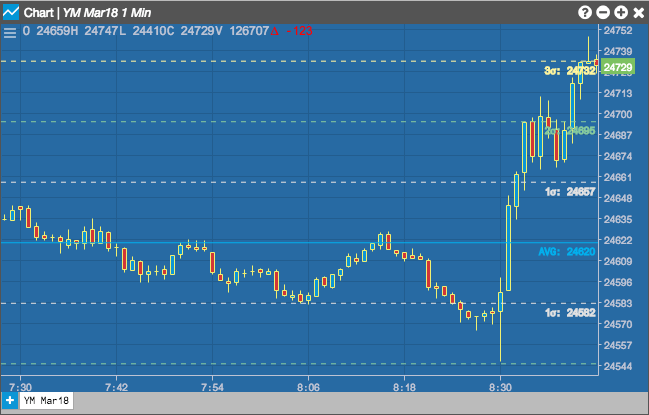Charts

# Valuation Lines

The Valuation Lines indicator displays a line representing the average of the visible prices in the chart. It also allows you to display lines for 1-3 standard deviations away from the average type.## Configuration Options• Field: Price or combination of prices to use as the base for average calculations. Possible values include:
• Open
• High
• Low
• Close
• HL/2 $$\left ( \frac{High + Low}{2} \right )$$
• HLC/3 $$\left ( \frac{High + Low + Close}{3} \right )$$
• HLCC/4 $$\left ( \frac{High + Low + Close + Close}{4} \right )$$
• OHLC/4 $$\left ( \frac{Open + High + Low + Close}{4} \right )$$
• Average Type: Type of average calculation to use:
• Mean
• Median
• Harmonic
• Display Average: Whether to display the average line.
• Display 1 Standard Deviation (1o): Whether to display the first standard deviation line.
• Display 2 Standard Deviation (2o): Whether to display the second standard deviation line.
• Display 2 Standard Deviation (3o): Whether to display the third standard deviation line.
• Color Selectors: Colors to use for graph elements.
• Display Axis Label: Whether to display the most recent value on the Y axis.

## Formula

VL = Average of Visbile Prices